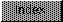```

NAME
IEEEDPFloor -- compute Floor function of IEEE double precision number

SYNOPSIS
x   = IEEEDPFloor(  y  );
d0/d1               d0/d1

double  x,y;

FUNCTION
Calculate the largest integer less than or equal to x and return it.
This value may have more than 32 bits of significance.

INPUTS
y -- IEEE double precision floating point value

RESULT
x -- IEEE double precision floating point value

BUGS